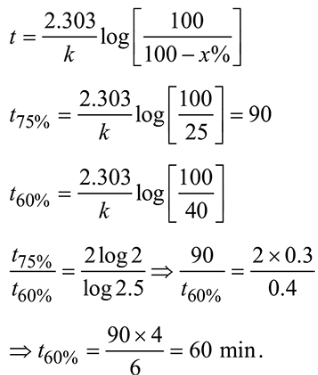# If 75 % of a first order reaction was completed in 90 minutes,

Question:

If $75 \%$ of a first order reaction was completed in 90 minutes, $60 \%$ of the same reaction would be completed in approximately (in minutes) ______________

(Take : $\log 2=0.30 ; \log 2.5=0.40$ )

Solution:

(60)